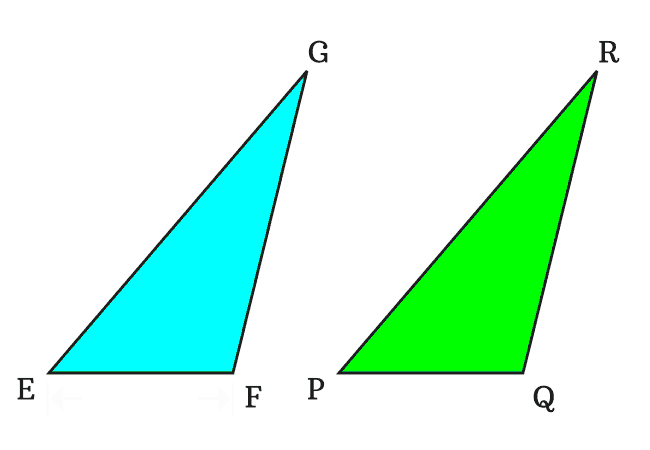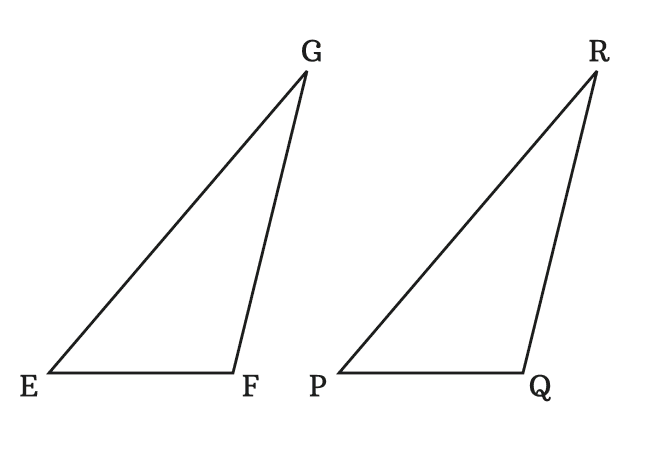# Congruent Triangles

The triangles that have equal corresponding angles and equal corresponding sides are called the congruent triangles.

## IntroductionThe congruence of any two triangles is determined by checking the following two geometrical factors.

1. The equality of corresponding sides of the triangles.
2. The equality of corresponding angles of the triangles.

If the two triangles have same properties, then one triangle is congruent to another triangle and vice-versa.

In general, the congruence of triangles can be understood easily by placing one triangle over another triangle. If the triangles are congruent, then every vertex of one triangle is exactly coincides with the corresponding vertex of another triangle. Similarly, the length of each side of one triangle is exactly coincides with the length of each side of another triangle.

### Example

$\Delta EFG$ and $\Delta PQR$ are two triangles. Let’s check the congruence of the both triangles geometrically.

#### Sides

In $\Delta EFG$, the three sides are $\overline{EF}$, $\overline{FG}$ and $\overline{GE}$. Similarly, $\overline{PQ}$, $\overline{QR}$ and $\overline{RP}$ are three sides of the $\Delta PQR$.The corresponding sides of $\overline{EF}$, $\overline{FG}$ and $\overline{GE}$ are $\overline{PQ}$, $\overline{QR}$ and $\overline{RP}$ respectively. Now, measure the length of each side by a ruler and then compare the lengths of corresponding sides of both triangles.

$(1).\,\,\,$ $EF \,=\, PQ \,=\, 3\,cm$

$(2).\,\,\,$ $FG \,=\, QR \,=\, 5\,cm$

$(3).\,\,\,$ $GE \,=\, RP \,=\, 6.4\,cm$

According to the lengths of both triangles, the lengths of corresponding sides of both triangles are congruent.

#### Angles

In $\Delta EFG$, the three interior angles are $\angle EFG$, $\angle FGE$ and $\angle GEF$. In $\Delta PQR$, the three angles are $\angle PQR$, $\angle QRP$ and $\angle RPQ$.The corresponding angles of $\angle EFG$, $\angle FGE$ and $\angle GEF$ are $\angle PQR$, $\angle QRP$ and $\angle RPQ$ respectively. Now, measure all three angles of each triangle by a protractor and compare the corresponding angles of the two triangles.

$(1).\,\,\,$ $\angle EFG \,=\, \angle PQR \,=\, 50^°$

$(2).\,\,\,$ $\angle FGE \,=\, \angle QRP \,=\, 103^°$

$(3).\,\,\,$ $\angle GEF \,=\, \angle RPQ \,=\, 27^°$

According to the comparison of corresponding angles of the two triangles $EFG$ and $PQR$, the corresponding angles of both triangles are also congruent.

##### Representation

The congruence of triangles is expressed in mathematics in a special way.

1. The triangle $EFG$ is congruent to the triangle $PQR$ and it is mathematically expressed as $\Delta EFG \cong \Delta PQR$.
2. Similarly, the triangle $PQR$ is congruent to the triangle $EFG$, and it is written as $\Delta PQR \cong \Delta EFG$ in mathematics.
Latest Math Topics
Jun 26, 2023
Jun 23, 2023

Latest Math Problems
Jul 01, 2023
Jun 25, 2023
###### Math Questions

The math problems with solutions to learn how to solve a problem.

Learn solutions

Practice now

###### Math Videos

The math videos tutorials with visual graphics to learn every concept.

Watch now

###### Subscribe us

Get the latest math updates from the Math Doubts by subscribing us.